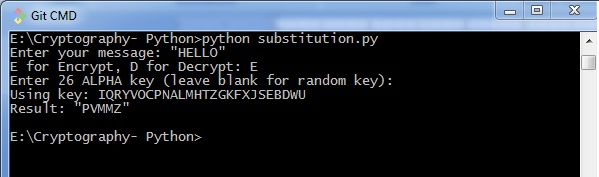# Simple Substitution Cipher

Simple substitution cipher is the most commonly used cipher and includes an algorithm of substituting every plain text character for every cipher text character. In this process, alphabets are jumbled in comparison with Caesar cipher algorithm.

## Example

Keys for a simple substitution cipher usually consists of 26 letters. An example key is −

plain alphabet : abcdefghijklmnopqrstuvwxyz
cipher alphabet: phqgiumeaylnofdxjkrcvstzwb


An example encryption using the above key is−

plaintext : defend the east wall of the castle
ciphertext: giuifg cei iprc tpnn du cei qprcni


The following code shows a program to implement simple substitution cipher −

import random, sys

LETTERS = 'ABCDEFGHIJKLMNOPQRSTUVWXYZ'
def main():
message = ''
if len(sys.argv) > 1:
with open(sys.argv, 'r') as f:
else:
message = raw_input("Enter your message: ")
mode = raw_input("E for Encrypt, D for Decrypt: ")
key = ''

while checkKey(key) is False:
key = raw_input("Enter 26 ALPHA key (leave blank for random key): ")
if key == '':
key = getRandomKey()
if checkKey(key) is False:
print('There is an error in the key or symbol set.')
translated = translateMessage(message, key, mode)
print('Using key: %s' % (key))

if len(sys.argv) > 1:
fileOut = 'enc.' + sys.argv
with open(fileOut, 'w') as f:
f.write(translated)
print('Success! File written to: %s' % (fileOut))
else: print('Result: ' + translated)

# Store the key into list, sort it, convert back, compare to alphabet.
def checkKey(key):
keyString = ''.join(sorted(list(key)))
return keyString == LETTERS
def translateMessage(message, key, mode):
translated = ''
charsA = LETTERS
charsB = key

# If decrypt mode is detected, swap A and B
if mode == 'D':
charsA, charsB = charsB, charsA
for symbol in message:
if symbol.upper() in charsA:
symIndex = charsA.find(symbol.upper())
if symbol.isupper():
translated += charsB[symIndex].upper()
else:
translated += charsB[symIndex].lower()
else:
translated += symbol
return translated
def getRandomKey():
randomList = list(LETTERS)
random.shuffle(randomList)
return ''.join(randomList)
if __name__ == '__main__':
main()


## Output

You can observe the following output when you implement the code given above −